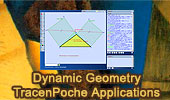# Dynamic Geometry: TracenPoche Interactive Geometry SoftwareTracenPoche Interactive Geometry Software Applications- Index TracenPoche is a free interactive or dynamic geometry software, written in Adobe Flash by the Sésamath association. It is widely used in French secondary schools.Archimedes Arbelos and Square 2. TracenPoche Interactive Geometry Software. Step-by-Step construction, Manipulation, and animation. Archimedes Arbelos and Square 1. TracenPoche Interactive Geometry Software. Step-by-Step construction, Manipulation, and animation. Bottema's Theorem. Invariance of an isosceles right triangle. Triangle and Squares with TracenPoche Interactive Geometry Software Step-by-Step construction, Manipulation, and animation. Complete Quadrilateral: Ortholine-Steiner Line. Step-by-Step construction, Manipulation, and animation TracenPoche Interactive Geometry Software. Eight-Point Circle Theorem Step-by-Step construction, Manipulation, and animation. TracenPoche Interactive Geometry Software. Feuerbach Points and Nine-Point Circle with TracenPoche interactive animation, manipulation, and step-by-step construction. Incenter, Incircle, Excircles. Four Circles Theorem Using TracenPoche Interactive Dynamic Software Step-by-Step construction, Manipulation, and animation. Interactive Gergonne Line and Nobbs Points. Tracenpoche Interactive Geometry Software. Step-by-Step construction, Manipulation, and animation. Incenter, Excenter, Incircle, Excircle. Using TracenPoche Dynamic Software Step-by-Step construction, Manipulation, and animation. Isogonic-Jacobi Theorem Using TracenPoche Dynamic Geometry Software Marion Walter's Theorem: Triangle and Hexagon areas Using TracenPoche Dynamic Geometry Software Morley's Triangle & Center: with TracenPoche interactive animation and manipulation. Newton's Theorem, Newton-Gauss Line Complete quadrilateral theorem. Using TracenPoche Tracenpoche Interactive Geometry Software, Online Step-by-Step construction, manipulation, and animation. Orthopole of a Triangle Using TracenPoche Dynamic Geometry Software Pappus Theorem. Using TracenPoche Interactive Geometry Software. Step-by-Step construction, Manipulation, and animation. Schiffler Point Four Euler Lines with TracenPoche interactive animation and manipulation. Simson Line. using Tracenpoche Interactive Geometry Software. Step-by-Step construction, Manipulation, and animation. Taylor Circle Theorem Using TracenPoche Dynamic Software Step-by-Step construction, Manipulation, and animation. Trapezoid, Triangle, Diagonals, Midpoints. Using Tracenpoche Interactive Geometry Software. Step-by-Step construction, Manipulation, and animation. Three Circles Theorem. Using TracenPoche Dynamic Software Triangle: Incircle, Perpendicular, Angle Bisector. Using Tracenpoche Interactive Geometry Software. Step-by-Step construction, Manipulation, and animation. Triangle, Medians, Six Circumcenters Concyclic. Using TracenPoche Interactive Geometry Software. Step-by-Step construction, Manipulation, and animation. Three Tangent Circles Theorem Using TracenPoche Dynamic Software Step-by-Step construction, Manipulation, and animation. Incenter of a triangle. Triangle and Squares Theorem 1 Using TracenPoche Interactive Geometry Software Step-by-Step construction, Manipulation, and animation.Home| Search | Geometry | Dynamic Geometry | Post a comment | Email | By Antonio Gutierrez